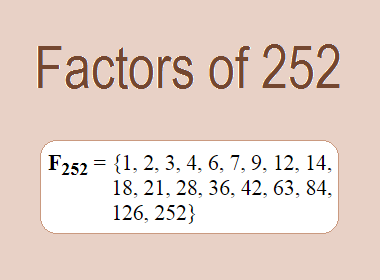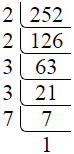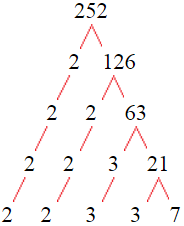# Factors of 252The factors of 252 are 1, 2, 3, 4, 6, 7, 9, 12, 14, 18, 21, 28, 36, 42, 63, 84, 126, and 252 i.e. F252 = {1, 2, 3, 4, 6, 7, 9, 12, 14, 18, 21, 28, 36, 42, 63, 84, 126, 252}. The factors of 252 are all the numbers that can divide 252 without leaving a remainder.

We can check if these numbers are factors of 252 by dividing 252 by each of them. If the result is a whole number, then the number is a factor of 252. Let's do this for each of the numbers listed above:

·        1 is a factor of 252 because 252 divided by 1 is 252.

·        2 is a factor of 252 because 252 divided by 2 is 126.

·        3 is a factor of 252 because 252 divided by 3 is 84.

·        4 is a factor of 252 because 252 divided by 4 is 63.

·        6 is a factor of 252 because 252 divided by 6 is 42.

·        7 is a factor of 252 because 252 divided by 7 is 36.

·        9 is a factor of 252 because 252 divided by 9 is 28.

·        12 is a factor of 252 because 252 divided by 12 is 21.

·        14 is a factor of 252 because 252 divided by 14 is 18.

·        18 is a factor of 252 because 252 divided by 18 is 14.

·        21 is a factor of 252 because 252 divided by 21 is 12.

·        28 is a factor of 252 because 252 divided by 28 is 9.

·        36 is a factor of 252 because 252 divided by 36 is 7.

·        42 is a factor of 252 because 252 divided by 42 is 6.

·        63 is a factor of 252 because 252 divided by 63 is 4.

·        84 is a factor of 252 because 252 divided by 84 is 3.

·        126 is a factor of 252 because 252 divided by 126 is 2.

·        252 is a factor of 252 because 252 divided by 252 is 1.

## How to Find Factors of 252?

1 and the number itself are the factors of every number. So, 1 and 252 are two factors of 252. To find the other factors of 252, we can start by dividing 252 by the numbers between 1 and 252. If we divide 252 by 2, we get a remainder of 0. Therefore, 2 is a factor of 252. If we divide 252 by 3, we get a remainder of 0. Therefore, 3 is also a factor of 252.

Next, we can check if 4 is a factor of 252. If we divide 252 by 4, we get a remainder of 0. Therefore, 4 is also a factor of 252. If we divide 252 by 5, we get a remainder of 2. Therefore, 5 is not a factor of 252. We can continue this process for all the possible factors of 252.

Through this process, we can find that the factors of 252 are 1, 2, 3, 4, 6, 7, 9, 12, 14, 18, 21, 28, 36, 42, 63, 84, 126, and 252. These are the only numbers that can divide 252 without leaving a remainder.

********************

********************

## Properties of the Factors of 252

The factors of 252 have some interesting properties. One of the properties is that the sum of the factors of 252 is equal to 728. We can see this by adding all the factors of 252 together:

1 + 2 + 3 + 4 + 6 + 7 + 9 + 12 + 14 + 18 + 21 + 28 + 36 + 42 + 63 + 84 + 126 + 252 = 728

Another property of the factors of 252 is that the prime factors of 252 are 2, 3, and 7 only.

## Applications of the Factors of 252

The factors of 252 have several applications in mathematics. One of the applications is in finding the highest common factor (HCF) of two or more numbers. The HCF is the largest factor that two or more numbers have in common. For example, to find the HCF of 252 and 84, we need to find the factors of both numbers and identify the largest factor they have in common. The factors of 252 are 1, 2, 3, 4, 6, 7, 9, 12, 14, 18, 21, 28, 36, 42, 63, 84, 126, and 252. The factors of 84 are 1, 2, 3, 4, 6, 7, 12, 14, 21, 28, 42, and 84. The largest factor that they have in common is 84. Therefore, the HCF of 252 and 84 is 84.

Another application of the factors of 252 is in prime factorization. Prime factorization is the process of expressing a number as the product of its prime factors. The prime factors of 252 are 2, 3, and 7 since these are the only prime numbers that can divide 252 without leaving a remainder. Therefore, we can express 252 as:

252 = 2 × 2 × 3 × 3 × 7

We can do prime factorization by division and factor tree method also. Here is the prime factorization of 252 by division method,252 = 2 × 2 × 3 × 3 × 7

Here is the prime factorization of 252 by the factor tree method,252 = 2 × 2 × 3 × 3 × 7

## Conclusion

The factors of 252 are the numbers that can divide 252 without leaving a remainder. The factors of 252 are 1, 2, 3, 4, 6, 7, 9, 12, 14, 18, 21, 28, 36, 42, 63, 84, 126, and 252. The factors of 252 have some interesting properties, such as having a sum of 728. The factors of 252 have several applications in mathematics, such as finding the highest common factor and prime factorization.